PMML Powered
 Company / Project Software PMML Producer PMML Consumer Supported Model TypeACTICO Rules PMML 3.0 through 4.2 Decision TreesAlpine PMML 4.1 Regression Models (Linear and Logistic) Naïve Bayes K-Means Alpine Forest SVM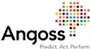KnowledgeSTUDIO PMML 3.2 Decision Trees Regression Models (Linear and Logistic) Neural Networks Clustering Models Rule Set Models (Scorecards) KnowledgeSEEKER PMML 3.2 Decision Trees StrategyBUILDER PMML 3.2 Decision Trees (Strategy Trees)BigML Public API PMML 4.1 Decision Trees (classification and regression)Model Integration Tool ™ PMML 4.2 PMML 4.2 Scorecards Regression Models (Linear and Logistic) Segmented Scorecard Models Decision Tree (Ensemble)Strategy Tree Optimization PMML 3.0, 3.1 PMML 3.0, 3.1 Decision Tree PowerCurve™ Strategy Management PMML 3.0, 3.1, 4.0, 4.1 PMML 3.0, 3.1, 4.0, 4.1 Decision Tree PMML 3.0, 3.1, 4.0, 4.1 Regression ModelModel Builder PMML 4.1, 3.2, 3.0 Scorecards Regression Models (Linear and Logistic) Neural Networks Decision Trees Segmented Scorecard Models Boosted Tree Ensembles All R PMML produced models (see R/Rattle entry below) Analytic Modeler Scorecard PMML 4.1 Scorecards Boosted Tree Ensembles Analytic Modeler Scorecard Professional PMML 4.2, 3.2 Scorecards Decision Optimizer PMML 3.2 PMML 3.2 Consumes: Scorecards Regression Models (Linear and Logistic) Neural Networks Decision Trees Produces: Decision Trees Blaze Advisor PMML 3.2, 4.2 Scorecards Regression Models (Linear and Logistic) Neural Networks Decision Trees Random Forests Model Executor for PMML PMML 2.0 through 4.2 Cluster General Regression Naïve Bayes Neural Networks Regression Ruleset Scorecard Trees Vector Machines Decision Management Platform Streaming PMML 2.0 through 4.2 Decision Trees Support Vector Machines Mining Models Neural Networks Regression & General Regression Clustering Models Naïve Bayes Ruleset Models Association Rules Scorecards Pre- and post-processing Text Mining Strategy Director PMML 3.2, 4.2 Scorecards Regression Models (Linear and Logistic)Financial Crime Risk Management Platform PMML 2.0 through 4.2 Decision Trees Support Vector Machines Mining Models Neural Networks Regression & General Regression Clustering Models Naïve Bayes Ruleset Models Association Rules Scorecards Pre- and post-processing Text Mining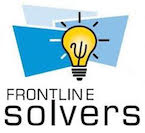XLMiner® SDK, Analytic Solver® Data Mining, AnalyticSolver.com PMML 4.2 PMML 4.2 Regression Models (Linear and Logistic) Decision Trees Neural Networks k-Nearest Neighbors Naïve Bayes Random Forest Time Series Association Rules (Producer Only) Transformations Multiple ModelsDataView360® Decision Engine PMML 3.0 through 4.3 General Regression Regression Neural Network Ruleset Trees Scorecards Vector Machine Multiple Models: Model Composition, Ensembles and SegmentationIBM SPSS Statistics 21 PMML4.0 PMML2.0 through 4.0 Clustering Models Decision Trees General Regression MiningModel Neural Networks Time Series Naïve Bayes (produced only by SPSS Statistics Server) Also consumes Ruleset, Regression, and Support Vector Machine Models IBM SPSS Statistics 22 PMML4.1 PMML2.0 through 4.1 Clustering Models Decision Trees General Regression k-Nearest Neighbors Mining Model Neural Networks Time Series Naïve Bayes (produced only by SPSS Statistics Server) Also consumes Ruleset, Regression, and Support Vector Machine Models IBM SPSS Statistics 23 PMML4.1 PMML2.0 through 4.1 Association Model (only produced) Clustering Models Decision Trees General Regression k-Nearest Neighbors Mining Model Neural Networks Time Series Naïve Bayes (produced only by SPSS Statistics Server) Also consumes Ruleset, Regression, and Support Vector Machine Models IBM SPSS Modeler 15 PMML4.0 PMML2.0 through 4.0 Produces: Association Model Clustering Models Decision Trees Mining Model Naïve Bayes Neural Networks Regression & General Regression Models Ruleset Models Support Vector Machines Consumes (scores): all of the above except Association, and Mining Model. IBM SPSS Modeler 16 PMML4.1 PMML2.0 through 4.1 Produces: Association Model Clustering Models Decision Trees k-Nearest Neighbors Mining Model Naïve Bayes Neural Networks Regression & General Regression Models Ruleset Models Support Vector Machines Consumes (scores): all of the above except Association, and Mining Model. IBM SPSS Modeler 17 PMML4.1 PMML2.0 through 4.1 Produces: Association Model Clustering Models Decision Trees k-Nearest Neighbors Mining Model Naïve Bayes Neural Networks Regression & General Regression Models Ruleset Models Support Vector Machines Consumes (scores): all of the above except Association. IBM SPSS Collaboration and Deployment Services v. 5.0 PMML2.0 through 4.0 Clustering Models Decision Trees Naïve Bayes Neural Networks Regression & General Regression Ruleset Models Support Vector Machines IBM SPSS Collaboration and Deployment Services v. 6.0 PMML2.0 through 4.1 Clustering Models Decision Trees Nearest Neighbors Naïve Bayes Neural Networks Regression & General Regression Ruleset Models Support Vector Machines IBM SPSS Collaboration and Deployment Services v. 7.0 PMML2.0 through 4.1 Clustering Models Decision Trees Nearest Neighbors Naïve Bayes Neural Networks Regression & General Regression Ruleset Models Support Vector Machines IBM Netezza Analytics v3.0 PMML 4.0 Association Rules Clustering Models (center-based and distribution-based) Decision Tree Models Naive Bayes IBM InfoSphere Warehouse V9.7 IBM InfoSphere Warehouse V10.1 IBM DB2 Advanced Enterprise Server Edition V10.5 IBM DB2 Advanced Workgroup Server Edition V10.5 PMML 3.2 PMML 2.0 through 3.2 Association Rules Models Sequence Models Naïve Bayes Models Logistic Regression Models PMML 3.0 Clustering Models (center and distribution based) Regression Models Decision Trees PMML 2.0 through 3.0 Clustering Models (center and distribution based) Regression Models Decision Trees Neural Networks KNIME 2.10 PMML 4.2 PMML 4.2 Neural Networks Regression and General Regression Models Clustering Models Decision Trees Naïve Bayes Rule Set Editor Rule Set Predictor Support Vector Machines including support for Transformation elements Model Ensembles And support for Transformation elements KNIME 2.10 with R extension PMML 4.1 PMML 4.1 All R PMML produced models (see R/Rattle entry below) that are compatible to PMML 4.1 KXEN Analytic Framework 4.0.10, 5.0.7 and 5.1.2 PMML 3.2 Regression Models, Clustering Models, Mining Models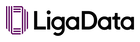Kamanja PMML 4.0 to 4.2 Assocation rules (Association) Cluster model (Clustering) General regression (Regression, Cox regression, Classification) k-Nearest neighbors (Regression, Classification, Clustering) Naive Bayes (Classification) Neural network (Regression, Classification (Except for the radialBasis value of the activationFunction attribute)) Regression (Regression, Classification) Rule set (Classification) Scorecard (Regression) Tree model (Classification) Support Vector Machine (SVM) (Regression, Classification) Ensemble model (Regression, Classification, Clustering) Microsoft SQL Server PMML 2.1 Decision Trees (Classification), Clustering Models (Distribution-based)MicroStrategy Data Mining Services Versions 8.0 and above PMML 3.0, 3.1, 4.0 PMML 2.0, 2.1, 3.0,3.1, 3.2, 4.0 Regression Models (Consumer & Producer) Decision Trees (Consumer & Producer) Mining Models (Consumer & Producer) Clustering Models (Consumer & Producer) Neural Network Models (Consumer Only) General Regression Models (Consumer Only) Support Vector Machine Models (Consumer Only) Rule Set Models (Consumer Only) Association Rules (Consumer & Producer) Time Series Models (Consumer & Producer)Lily PMML 2.0 through 4.2 Decision Trees Support Vector Machines Mining Models Neural Networks Regression & General Regression Clustering Models Naïve Bayes Ruleset Models Association Rules Scorecards Pre- and post-processing Text MiningPyMC3.BN PMML 4.3 PMML 4.3 Bayesian Networks Matlab GP PMML 4.3 PMML 4.3 Gaussian Process Regression Pmml-scoring-engine PMML 4.3 Gaussian Process RegressionAugustus 0.4x PMML 4.0 PMML 4.0 Decision Trees Regression Naïve Bayes Baseline (With Segmentation on all of the above) Augustus 0.5x PMML 4.1 PMML 4.1 Baseline Clusters Naïve Bayes Regression RuleSet Trees (With Segmentation on all of the above) Augustus 0.6x PMML 4.1 PMML 4.1 Baseline Clusters Trees (With Segmentation on all of the above)Java Class Model API for PMML (JPMML-Model) PMML 3.0 through 4.2 All model types Java Evaluator API for PMML (JPMML-Evaluator) PMML 3.0 through 4.2 Association Rules Decision Trees Clustering General Regression Mining Model Naïve Bayes k-NN Neural Network Regression Rule Sets Scorecards SVM Openscoring REST API PMML 3.0 through 4.2 Association Rules Decision Trees Clustering General Regression Mining Model Naïve Bayes k-NN Neural Network Regression Rule Sets Scorecards SVMSignal Hub 2.7 PMML 4.2 PMML 3.0 through 4.2 Produces Decision Trees Clustering Regression Models Naïve Bayes Support Vector Machines Neural Networks Consumes Association Rules Decision Trees Clustering General Regression Mining Model Naïve Bayes Neural Networks Regression Models Rule Sets Scorecards Support Vector Machines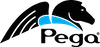Pega Platform 7.3.1 PMML 3.0 through 4.3 Cluster model General regression Mining (multiple/ensemble) model Neural Network k-Nearest Neighbors Naïve Bayes Ruleset Regression Support Vector Machine Scorecard Tree Pervasive DataRush Pervasive DataRush V5.0 PMML 3.2 PMML 3.2 Decision Trees (Consumer & Producer) Regression Models (Consumer & Producer) Clustering Models (kmeans Center-Based) Association Rules (Producer) Naïve Bayes (Consumer & Producer)PMML Connexion PMML 2.0 through 4.0 Decision Trees Support Vector Machines Neural Networks Regression and General Regression Clustering Models Naiive BayesRapidMiner with PMML Extension PMML 3.2, 4.0 Linear Regression Logistic Regression Models Decision Trees Rules K-Medoids K-Means Naïve Bayes R PMML package PMML 4.3 kNN Mining Models Regression Models General Regression Models (including Cox) Neural Networks Decision Trees Clustering Models Association Rules Support Vector Machines Multinomial Logistic Regression Random Forest Random Survival Forest Naïve Bayes Classifiers And support for merging of Transformation elements PMMLTransformations Package PMML 4.3 Transformations Salford Systems CART 6.0 PMML 3.1 Decision Trees Composition TreeNet 2.0 Pro 3.1 Composition Mars 3.0 3.2 Simple Regression (uses transformations and user-defined functions to implement basis functions) SAND Technology SAND CDBMS V6.1 PMML extension PMML 3.1, 3.2 Association Rules Clustering Models Regression Models Neural Networks Naïve Bayes Support Vector Machines Ruleset Model Decision TreesSAS Enterprise Miner PMML 2.1 (EM 5.1) PMML 3.1 (EM 5.3) PMML 4.0 (EM 7.1) PMML 4.1 (EM 12.3 and 13.1) Linear Regression Logistic Regression Decision Trees Neural Networks Clustering Association Rules SAS Base 9.3M2 PMML 4.0 Clustering Neural Networks Regression Tree SAS Base 9.40 PMML 4.1 Clustering Neural Networks Regression Tree Scorecard General Regression SAS Base V9.40M1 PMML 4.1 Clustering Neural Networks Regression Tree Scorecard General Regression Naïve Bayes Support Vector Machine Time Series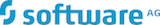Apama Streaming Analytics PMML 2.0 through 4.2 Decision Trees Support Vector Machines Mining Models Neural Networks Regression & General Regression Clustering Models Naïve Bayes Ruleset Models Association Rules Scorecards Pre- and post-processing Text MiningApache Spark PMML 4.2 Clustering Models K-Means Regression Models (Linear, Ridge, Binary Logistic) Lasso Model SVMSparkling Logic SMARTS™ PMML 4.2 Neural Networks Support Vector Machines Trees / Decision Trees Naïve Bayes Regression (Linear, Logistic, Multinomial) & General Regression Clustering Models Ruleset Models Scorecards Mining Models (incl. Random Forest) Transformations, Pre-post-processingTeradata Warehouse Miner v5.3.1 PMML 2.1 through 3.2 Regression Models, Decision Trees, Neural Networks, Clustering Models (center-based), Mining Models (regression types only)TIBCO Spotfire 6.5 PMML 4.1 All R PMML produced models (see R/Rattle entry above) TIBCO Enterprise Runtime for R 2.5 PMML 4.1 All R PMML produced models (see R/Rattle entry above) Weka (Pentaho) Weka PMML 3.2 Regression and General Regression Neural Networks Rule Set Models Decision TreesPMML 4.3 Decision Trees Random Forest Models Clustering Regression Models Zementis Predictive Analytics Engine PMML 2.0 through 4.3 Decision Trees Support Vector Machines Mining Models Neural Networks Regression & General Regression Clustering Models Naïve BayesRuleset Models Association RulesScorecards Pre- and post-processing Text Mining Universal PMML Plug-in for Hadoop PMML 2.0 through 4.3 Decision Trees Support Vector Machines Mining Models Neural Networks Regression & General Regression Clustering Models Naïve BayesRuleset Models Association RulesScorecards Pre- and post-processing Text Mining
 e-mail info at dmg.org